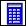> > Using the calculator

Using the calculator
To call the calculator, click Analysis and then, in the Utilities group, click(Calculator). Depending on the option selected in the System Settings dialog box (click File > Settings > System), Creo Elements/Direct Modeling displays either a standard calculator (the default) or an RPN (Reverse Polish Notation) calculator.
The calculator provides the ability to measure 3D and 2D geometry and to do a variety of math calculations using these measurements or other user-defined figures. Numerical values can be cut and pasted to/from other Creo Elements/Direct Modeling commands to save retyping.
The stack of registers starts with x at the bottom and goes up through y, z and finally to the t register. Each register (colored dark blue) contains a single numeric value.
The calculator:
Measures distances, radii, angles and other 2D and 3D properties using a variety of measurement options. Results of the measurement are displayed and saved as numeric values in the calculator menu for further operations.
Converts between inch and metric values.
Provides both basic (add, subtract, multiply, divide) and advanced math functions (trigonometric, logarithmic, etc).
Stores and recalls numeric values.
Allows calculated values to be used as input for other Creo Elements/Direct Modeling commands. These can be scalar or 2D or 3D vector values.
Accepts input values from the keyboard or the graphical number pad of the calculator menu.
Provides the ability to manipulate the numeric stack. This includes: rolling the stack up and down, switching X and Y values, clearing the X value or the entire stack, and editing the numbers in the stack.
How to use the calculator
Move your cursor to any of the cells and type numbers to enter, or modify, the value that is displayed in the cell. The light blue x,y,z and t buttons on the left put the register value into other Creo Elements/Direct Modeling commands. The xy and xyz buttons on the right put 2D (x,y) or 3D (x,y,z) vector values into other Creo Elements/Direct Modeling commands.
Use the light blue mm->in and in->mm keys to convert the X register value to the desired unit.
Use the blue number tablet (keys 0-9) to enter new values for calculation.
Use the green Sto Rcl key with any of the blue number keys (0-9) to store and recall the value of the X register.
Use the green R keys to scroll the numeric stack up or down.
Use the green X<>Y key to switch the contents of the X and Y registers.
Use the red Clx key to clear just the X register, the red Cl key to clear all of the registers, or the red <- key to delete the last digit from the X register.
Use the yellow Enter key to save the register contents.
Use the dark blue + - * / keys for addition, subtraction, multiplication and division.
Use the scroll bar to see the additional math functions. There are several groups of additional math functions:
Dark Blue (measure) - shown by default
Yellow (trigonometry)
Green (logarithm)
Light Blue (polar/rectangular conversion and others)
The calculator does not measure infinite construction geometry.